Class 9 NCERT Solutions- Chapter 7 Triangles – Exercise 7.1

• Last Updated : 28 Dec, 2020

Question 1. In quadrilateral ACBDAC = AD and AB bisects ∠ A (see Fig. 7.16). Show that ∆ ABC ≅ ∆ ABD What can you say about BC and BD?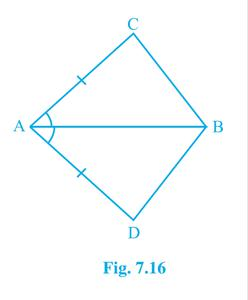Solution:

Given that AC and AD are equal

i.e. AC = AD and the line AB bisects ∠A.

Considering the two triangles ΔABC and ΔABD,

Where,

AC = AD { As given}………………………………………… (i)

∠CAB = ∠DAB ( As AB  bisects of ∠A)……………. (ii)

AB { Common side of both the triangle} …….. …(iii)

From above three equation both the triangle satisfies “SAS” congruency criterion

So, ΔABC ≅ ΔABD.

Also,

BC and BD will be of equal lengths as they are corresponding parts of congruent triangles(CPCT).

So BC = BD.

Question 2. ABCD is a quadrilateral in which AD = BC and ∠ DAB = ∠ CBA (see Fig. 7.17). Prove that

(i) ∆ ABD ≅ ∆ BAC

(ii) BD = AC

(iii) ∠ ABD = ∠ BAC.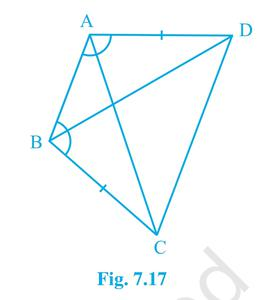Solution:

(i) Given that AD = BC,

And ∠ DAB = ∠ CBA.

Considering two triangles ΔABD and ΔBAC.

Where,

AD = BC { As given }………………………………………….. (i)

∠ DAB = ∠ CBA { As given also}……………………….. (ii)

AB  {Common side of both the triangle)…………. (iii)

From above three equation two triangles ABD and BAC satisfies “SAS” congruency criterion

So, ΔABD ≅ ΔBAC

(ii) Also,

BD and AC  will be equal as they are corresponding parts of congruent triangles(CPCT).

So BD = AC

(iii) Similarly,

∠ABD and ∠BAC will be equal as they are corresponding parts of congruent triangles(CPCT).

So,

∠ABD = ∠BAC.

Question 3. AD and BC are equal perpendiculars to a line segment AB (see Fig. 7.18). Show that CD bisects AB.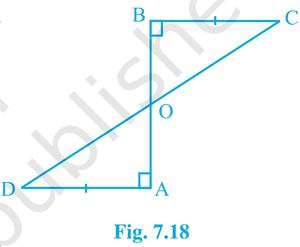Solution:

Given that AD and BC are two equal perpendiculars to a line segment AB

Considering two triangles ΔAOD and ΔBOC

Where,

∠ AOD = ∠ BOC {Vertically opposite angles}………………. (i)

∠ OAD = ∠ OBC {Given that they are perpendiculars}…. (ii)

AD = BC {As given}………………………………………………… (iii)

From above three equation both the triangle satisfies “AAS” congruency criterion

So, ΔAOD ≅ ΔBOC

AO and BO will be equal as they are corresponding parts of congruent triangles(CPCT).

So, AO = BO

Hence, CD bisects AB at O.

Question 4. l and m are two parallel lines intersected by another pair of parallel lines p and q (see Fig. 7.19). Show that ∆ ABC ≅ ∆ CDA.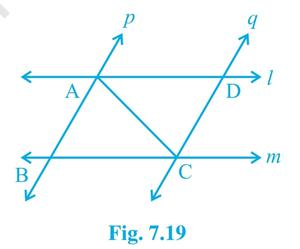Solution:

Given that l and m are two parallel lines p and q are another pair of parallel lines

Considering two triangles ΔABC and ΔCDA

Where,

∠ BCA = ∠DAC  {Alternate interior angles}…. (i)

∠ BAC = ∠ DCA {Alternate interior angles}…. (ii)

AC  {Common side of two triangles}………….(iii)

From above three equation both the triangle satisfies “ASA” congruency criterion

So, ΔABC ≅ ΔCDA

Question 5. Line l is the bisector of an angle ∠ A and B is any point on l. BP and BQ are perpendiculars from B to the arms of ∠A (see Fig. 7.20). Show that:

(i) ∆ APB ≅ ∆ AQB

(ii) BP = BQ or B is equidistant from the arms of ∠ A.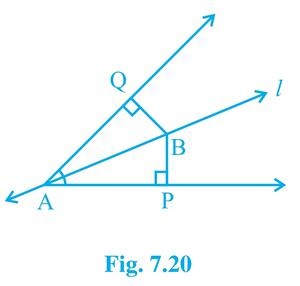Solution:

Given that, Line l is the bisector of an angle ∠ A and B

BP and BQ are perpendiculars from angle A.

Considering two triangles ΔAPB and ΔAQB

Where,

∠ APB = ∠ AQB { Two right angles as given }…… (i)

∠BAP = ∠BAQ (As line l  bisects  angle A }……… (ii)

AB  { Common sides of both the triangle }……… (iii)

From above three equation both the triangle satisfies “AAS” congruency criterion

So, ΔAPB≅ ΔAQB.

(ii) Also we can say BP and BQ are equal as they are corresponding parts of congruent triangles(CPCT).

So, BP = BQ

Question 6. In Fig. 7.21, AC = AE, AB = AD and ∠ BAD = ∠ EAC. Show that BC = DE.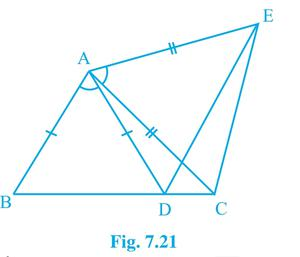Solution:

Given that AC = AE, AB = AD

As given that ∠BAD = ∠EAC

Adding ∠DAC on both the sides

We get,

∠BAD + ∠DAC = ∠EAC + ∠ DAC

Considering two triangles ΔABC and ΔADE

Where,

AC = AE  { As given }…………………… (i)

∠BAC = ∠EAD { Hence proven }…….. (ii)

AB = AD {As also given }……………….. (iii)

From above three equation both the triangle satisfies “SAS” congruency criterion

(ii) Also we can say BC and  DE are equal as they are corresponding parts of congruent triangles(CPCT).

So that BC = DE.

Question 7. AB is a line segment and P is its mid-point. D and E are points on the same side of AB such that ∠ BAD = ∠ ABE and ∠ EPA = ∠ DPB (see Fig. 7.22). Show that:

(i) ∆ DAP ≅ ∆ EBP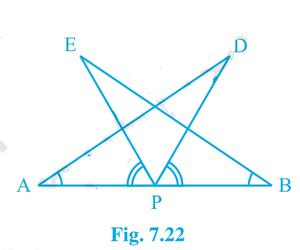Solution:

Given that P is the mid-point of line  AB, So AP = BP

Also, ∠ BAD = ∠ ABE and ∠ EPA = ∠ DPB

Now adding ∠DPE on both the sides of two equal angle ∠ EPA = ∠ DPB

∠ EPA + ∠ DPE = ∠ DPB + ∠DPE

Which implies that two angles ∠ DPA = ∠ EPB

Considering two triangles ∆ DAP and ∆ EBP

∠ DPA = ∠ EPB { Hence proven }…… (i)

AP = BP { Hence Given }……………… (ii)

∠ BAD = ∠ ABE { As given }…………..(iii)

From above three equation both the triangle satisfies “ASA” congruency criterion

So, ΔDAP ≅ ΔEBP

(ii) Also we can say AD and BE are equal as they are corresponding parts of congruent triangles(CPCT).

Question 8. In right triangle ABC, right angled at C, M is the mid-point of hypotenuse AB. C is joined to M and produced to a point D such that DM = CM. Point D is joined to point B (see Fig. 7.23). Show that:

(i) ∆ AMC ≅ ∆ BMD

(ii) ∠ DBC is a right angle.

(iii) ∆ DBC ≅ ∆ ACB

(iv) CM =  1/2 AB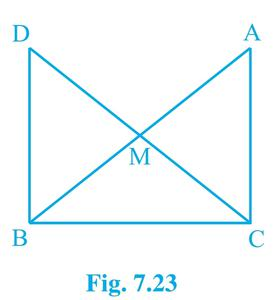Solution:

Given that M is the mid-point of  AB

So AM = BM

∠ ACB = 90°

and DM = CM

(i) Considering two triangles ΔAMC and ΔBMD:

AM = BM { As given }………………………………………. (i)

∠ CMA = ∠ DMB { Vertically opposite angles }…. (ii)

CM = DM { As given also}……………………………….. (iii)

From above three equation both the triangle satisfies “SAS” congruency criterion

So, ΔAMC ≅ ΔBMD

(ii) From above congruency we can say

∠ ACD = ∠ BDC

Also alternate interior angles of two parallel lines AC and DB.

Since sum of two co-interiors angles results to 180°.

So, ∠ ACB + ∠ DBC = 180°

∠ DBC = 180° – ∠ ACB

∠ DBC = 90° { As ∠ACB =90° }

(iii) In ΔDBC and ΔACB,

BC  { Common side of both the triangle }……. (i)

∠ ACB = ∠ DBC { As both are right angles }….(ii)

DB = AC (by CPCT)………………………………….. (iii)

From above three equation both the triangle satisfies “SAS” congruency criterion

So, ΔDBC ≅ ΔACB

(iv) As M is the mid point so we can say

DM = CM = AM = BM

Also we can say that AB = CD ( By CPCT )

As M is the mid point of CD we can write

CM + DM = AB

Hence, CM + CM = AB  (As DM = CM )

Hence, CM = (½) AB

My Personal Notes arrow_drop_up